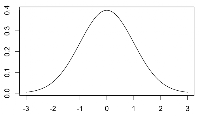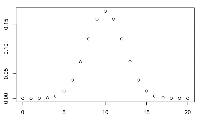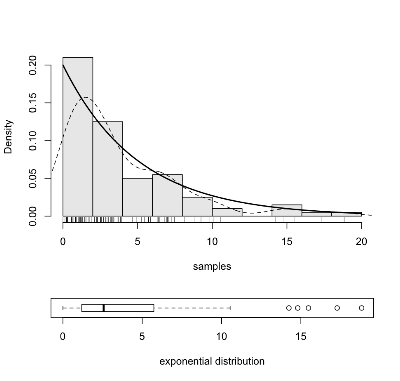## Tuesday, February 08, 2011

### Using R for Introductory Statistics, Chapter 5, Probability Distributions

In Chapter 5 of Using R for Introductory Statistics we get a brief introduction to probability and, as part of that, a few common probability distributions. Specifically, the normal, binomial, exponential and lognormal distributions make an appearance.

For each distribution, R provides four functions whose names start with the letters d, p, q or r followed by the family name of the distribution. For example, rnorm produces random numbers drawn from a normal distribution. The letters stand for:

 d density/mass function p probability (cumulative distribution function) P(X <= x) q quantiles, given q, the smallest x such that P(X <= x) > q r random number generation

#### Normal

The Gaussian or normal distribution has a prominent place due to the central limit theorem. It is widely used to model natural phenomena like variations in height or weight as well as noise and error. The 68-95-99.7 rule says:• 68% of the data falls within 1 standard deviation of the mean
• 95% of the data falls within 2 standard deviations of the mean
• 99.7% of the data falls within 3 standard deviations of the mean

To plot a normal distribution, define some points x, and use dnorm to generate the density at those points.

```x <- seq(-3,3,0.1)
plot(x=x, y=dnorm(x, mean=0, sd=1), type='l')
```

#### BinomialA Bernoulli trial is an experiment which can have one of two possible outcomes. Independent repeated Bernoulli trials give rise to the Binomial distribution, which is the probability distribution of the number of successes in n independent Bernoulli trials. Although the binomial distribution is discrete, in the limit as n gets larger, it approaches the normal distribution.

```x <- seq(0,20,1)
plot(x=x, y=dbinom(x,20,0.5))
```

#### Uniform

A Uniform distribution just says that all allowable values are equally likely, which comes up in dice or cards. Uniform distributions come in either continuous or discrete flavors.

#### Log-normal

The log-normal distribution is a probability distribution of a random variable whose logarithm is normally distributed. If X is a random variable with a normal distribution, then Y = exp(X) has a log-normal distribution. Analogously to the central limit theorem, the product of many independent random variables multiplied together tends toward a lognormal distribution. It can be used to model continuous random quantities whose distribution is skewed and non-negative, for example income or survival.

```samples <- rlnorm(100, meanlog=0, sdlog=1)
par(fig=c(0,1,0,0.35))
boxplot(samples, horizontal=T, bty="n", xlab="log-normal distribution")
par(fig=c(0,1,0.25,1), new=T)
s <- seq(0,max(samples),0.1)
d <- dlnorm(s, meanlog=0, sdlog=1)
hist(samples, prob=T, main="", col=gray(0.9), ylim=c(0,max(d)))
lines(density(samples), lty=2)
rug(samples)
```#### Exponential

The exponential distribution is used to model the time interval between successive random events such as time between failures arising from constant failure rates. The following plot is generated by essentially the same code as above.